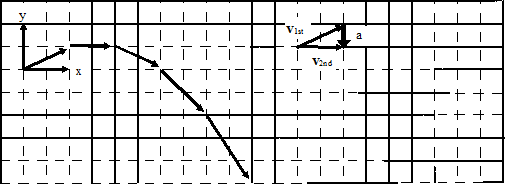# 02. Drawing Motion Diagrams

$$\newcommand{\vecs}{\overset { \rightharpoonup} {\mathbf{#1}} }$$ $$\newcommand{\vecd}{\overset{-\!-\!\rightharpoonup}{\vphantom{a}\smash {#1}}}$$$$\newcommand{\id}{\mathrm{id}}$$ $$\newcommand{\Span}{\mathrm{span}}$$ $$\newcommand{\kernel}{\mathrm{null}\,}$$ $$\newcommand{\range}{\mathrm{range}\,}$$ $$\newcommand{\RealPart}{\mathrm{Re}}$$ $$\newcommand{\ImaginaryPart}{\mathrm{Im}}$$ $$\newcommand{\Argument}{\mathrm{Arg}}$$ $$\newcommand{\norm}{\| #1 \|}$$ $$\newcommand{\inner}{\langle #1, #2 \rangle}$$ $$\newcommand{\Span}{\mathrm{span}}$$ $$\newcommand{\id}{\mathrm{id}}$$ $$\newcommand{\Span}{\mathrm{span}}$$ $$\newcommand{\kernel}{\mathrm{null}\,}$$ $$\newcommand{\range}{\mathrm{range}\,}$$ $$\newcommand{\RealPart}{\mathrm{Re}}$$ $$\newcommand{\ImaginaryPart}{\mathrm{Im}}$$ $$\newcommand{\Argument}{\mathrm{Arg}}$$ $$\newcommand{\norm}{\| #1 \|}$$ $$\newcommand{\inner}{\langle #1, #2 \rangle}$$ $$\newcommand{\Span}{\mathrm{span}}$$$$\newcommand{\AA}{\unicode[.8,0]{x212B}}$$

Motion diagrams are of crucial importance in investigating scenarios involving multi-dimensional motion. For example, analyze the following scenario:

In the shot put, a large mass is thrown at an angle of 22oabove horizontal, from a position of 2 m above the ground, a horizontal distance of 25 m.

A motion diagram for this scenario is sketched below.• Horizontal (x) and vertical (y) coordinate systems are clearly indicated.
• The acceleration is determined by the same method as in one-dimensional motion. In this case, the acceleration was determined near the beginning of the motion. Determining the acceleration at any other time will also indicate that its direction is straight downward
• In constructing the motion diagram, only a portion of the entire motion of the shot put is illustrated. For this motion diagram, analysis will begin the instant after the shot put leaves the putter’s hand, and analysis will end the instant before the shot put hits the ground. It is of extreme importance to clearly understand the beginning and the end of the motion that you will describe. The acceleration of the shot put while in the putter’s hand, and the acceleration upon contact with the ground, has been conveniently left out of this analysis. Unless explicit information is either provided or desired about these accelerations, it is best to focus analysis on the simplest essentials of the motion.

This page titled 02. Drawing Motion Diagrams is shared under a CC BY-NC-SA license and was authored, remixed, and/or curated by Paul D'Alessandris.